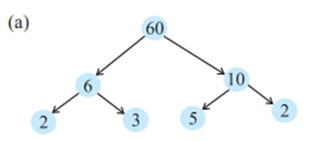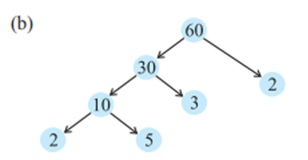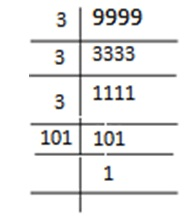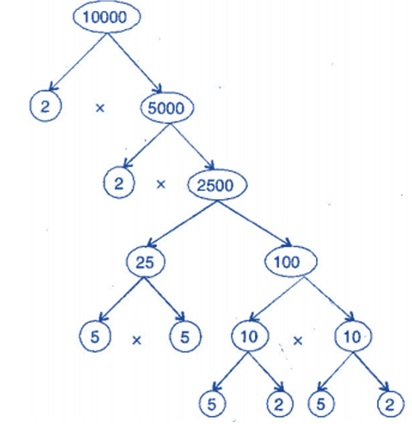Study Materials

# NCERT Solutions for Class 6th Mathematics

Page 5 of 7

## Chapter 3. Playing with Numbers

### Exercise 3.5

Exercise 3.5

1. Which of the following statements are true?

(a) If a number is divisible by 3, it must be divisible by 9.

(b) If a number is divisible by 9, it must be divisible by 3.

(c) A number is divisible by 18, if it is divisible by both 3 and 6.

(d) If a number is divisible by 9 and 10 both, then it must be divisible by 90.

(e) If two numbers are co-primes, at least one of them must be prime.

(f) All numbers which are divisible by 4 must also be divisible by 8.

(g) All numbers which are divisible by 8 must also be divisible by 4.

(h) If a number exactly divides two numbers separately, it must exactly divide their sum.

(i) If a number exactly divides the sum of two numbers, it must exactly divide the two numbers separately.

2. Here are two different factor trees for 60. Write the missing numbers.

Solution:

There are two different way as follow:3. Which factors are not included in the prime factorization of a composite number?

Solution: 1 and the composite number itself not included in the prime factorization of a composite number.

4. Write the greatest 4-digit number and express it in terms of its prime factors.

Solution: The greatest 4-digit number -5. Write the smallest 5-digit number and express it in the form of its prime factors.

Solution:

The smallest five diigit number is 10000.

It's tree factor is :Hence the prime factorisation =

2 × 2 × 2 × 2 × 5 × 5 × 5 × 5

6. Find all the prime factors of 1729 and arrange them in ascending order. Now state the relation, if any; between two consecutive prime factors.

Solution:

Prime factors of 1729 are 7 × 13 × 19.7. The product of three consecutive numbers is always divisible by 6. Verify this statement with the help of some examples.

Solution:  Among the three consecutive numbers, there must be one even number and one multiple of 3. Thus, the product must be multiple of 6.

Example:

(i) 2 × 3 × 4 = 24

(ii) 4 × 5 × 6 = 120

8. The sum of two consecutive odd numbers is divisible by 4. Verify this statement with the help of some examples.

Solution: 3 + 5 = 8 and 8 is divisible by 4.

5 + 7 = 12 and 12 is divisible by 4.

7 + 9 = 16 and 16 is divisible by 4.

9 + 11 = 20 and 20 is divisible by 4.

9. In which of the following expressions, prime factorisation has been done?Solution:  In expressions (b) and (c), prime factorization has been done.

10. Determine if 25110 is divisible by 45.

[Hint: 5 and 9 are co-prime numbers. Test the divisibility of the number by 5 and 9].

Solution: The prime factorization of 45 = 5 × 9 25110 is divisible by 5 as ‘0’ is at its unit place.

25110 is divisible by 9 as sum of digits is divisible by 9.

Therefore, the number must be divisible by 5 × 9 = 45

11. 18 is divisible by both 2 and 3. It is also divisible by 2 × 3 = 6. Similarly, a number is divisible by both 4 and 6. Can we say that the number must also be divisible by 4 × 6 = 24? If not, give an example to justify your answer.

Solution: No. Number 12 is divisible by both 6 and 4 but 12 is not divisible by 24.

12. I am the smallest number, having four different prime factors. Can you find me?

Solution: The smallest four prime numbers are 2, 3, 5 and 7.

Hence, the required number is 2 × 3 × 5 × 7 = 210

Page 5 of 7

Chapter Contents: